Binary adder is one of the basic combinational logic circuits. The outputs of a combinational logic circuit depend on the present input only. In other words, outputs of combinational logic circuit do not depend upon any previously applied inputs. It does not require any memory like component. Binary adder is one of the basic combinational logic circuits as present state of input variables.

Before designing a binary adder, let us know some basic rules of binary addition. The most basic binary addition is addition of two single bit binary numbers that is addition of two binary digits.

The binary digits are 0 and 1. Hence, there must be four possible combinations of binary addition of two binary bits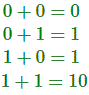In the above list, first three binary operations result in one bit but fourth one result in two bits. In one bit binary addition, if augend and addend are 1, the sum will have two digits. The higher significant bit (HSB) or Left side bit is called carry and the list significant bit (LSB) or right side bit of the result is called sum bit. The logical circuit performs this one bit binary addition is called half adder.

For designing a half adder logic circuit, we first have to draw the truth table for two input variables i.e. the augend and addend bits, two outputs variables carry and sum bits.
In first three binary additions, there is no carry hence the carry in these cases are considered as 0.

#### Truth Table for Half Adder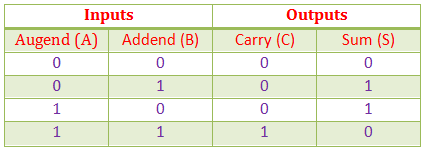Now from this truth table we can draw K-map for carries and sums separately.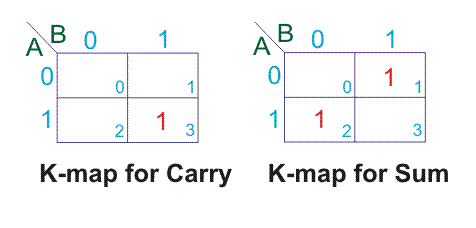For above K-maps we get,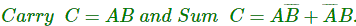Hence, the logical design of Half Adder would be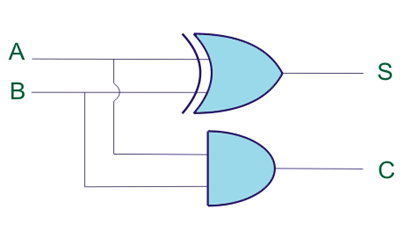Although from truth table it is clearly seen that carry (C) column signifies AND operation and sum (S) column signifies XOR operation between input variables but till we went through K-map as it is general practice to do so for more complex binary logic operations.

Before knowing about full adder, let us know what is full addition? For that let us consider the example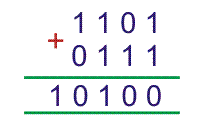There, are two four bits binary numbers 1101 and 0111 which we have to add. The process of binary addition is like follows,

1. We have to add first list significant bit (LSB) of both 4bits binary number first and this will result a two bits binary number.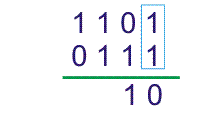Here, LSB of 1101 and 0111 are 1, hence 1 + 1 = 10. The LSB of 10 is 0 and higher significant bit (HSB) is 1.
2. The LSB of the result is sum and to be put at the list significant position of the final result of the sum, and HSB of the two bits results will be carry and to be added with next higher significant bit of two 4bits augend and addend are 0 and 1 and the carry of previous result i.e. 1 to be added with 0 and 1.
3.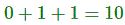4. After this addition, that is next higher than list significant bit of bits of both binary augend and addend and it is previous carry we get another two bits result. This also has carry and sum. Here also we will write sum at final result and add the carry to the next higher significant bits of augend and addend. This will continue up to most significant bit of augend and addend.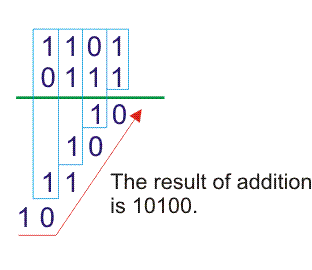Full adder is a conditional circuit which performs full binary addition that means it adds two bits and a carry and outputs a sum bit and a carry bit.
Any bit of augend can either be 1 or 0 and we can represent with variable A, similarly any bit of addend we represent with variable B. The carry after addition of same significant bit of augend and addend can represent by C. Hence truth table for all combinations of A, B and C is as follows,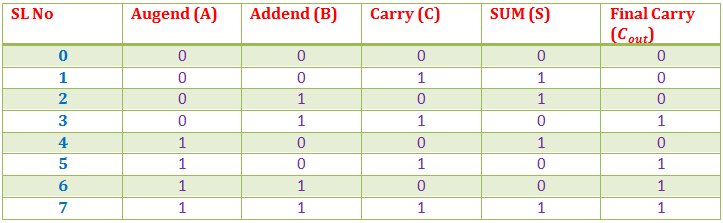From the above table, we can draw K-map for sum (s) and final carry (Cout).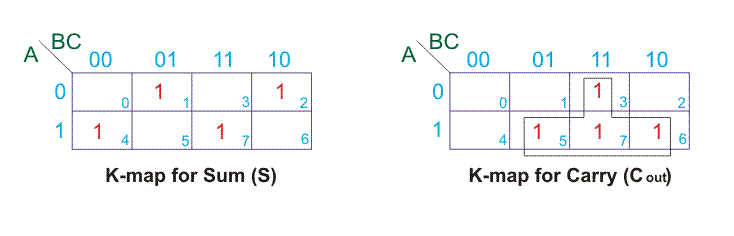Hence, from K-maps,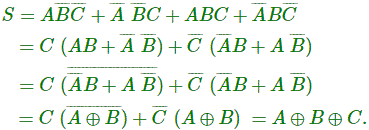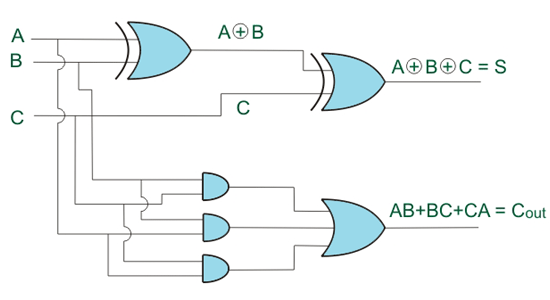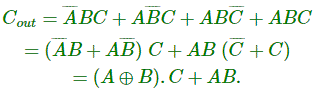The inter connection of 4 full adder in 4bit parallel adder is shown below,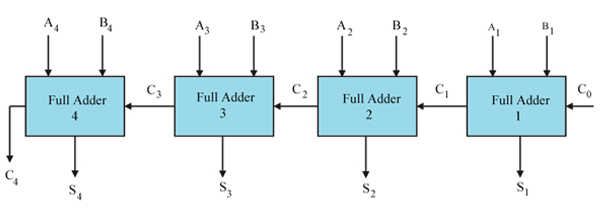Let us examine the justification of the above circuit by taking an example of addition of two 4 bit binary numbers.
Let us add 1011 with 1101.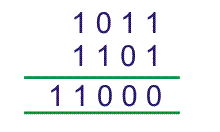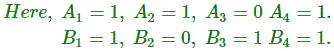As there is no previous carry C0 = 0.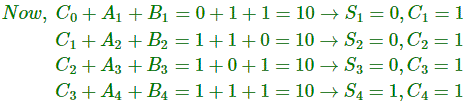Therefore, final result of the addition would be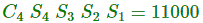There are 1 bit, 2 bits and 4 bits parallel adders ICs commercially available in market. For n bit parallel adder required number of such ICs are connected together.
4 bit parallel adder IC is 4008. In n bit parallel adder, output carry terminal of one IC would be connected with input carry terminal of next IC.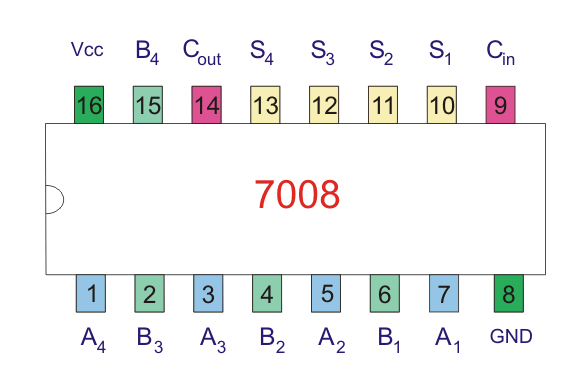Want To Learn Faster? 🎓
Get electrical articles delivered to your inbox every week.
No credit card required—it’s 100% free.